## Example Questions

### Example Question #1 : How To Multiply Fractions

There are 340 students at Saint Louis High School in the graduating senior class. Of these students, 9/10 are going to college.  Of those going to college, 2/5 are going to Saint Louis University. How many students are going to Saint Louis University?

103

122

306

136

The answer cannot be determined from the given information.

122

Explanation:

122 students are going to Saint Louis University. To answer this question, the following equation can be used: 340*(9/10)*(2/5) .  This is then rounded down to 122 students attending Saint Louis University.

### Example Question #1 : Operations

If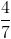of a number is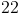, what is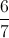of that number?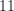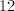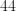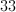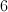Explanation:

The least common multiple of 4 and 6 is 12.

So we know ifof the number isthen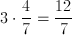of the number is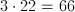.

So then it follows that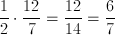of the number is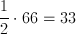.

### Example Question #3 : How To Multiply Fractions

If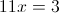and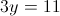, what is the value of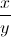?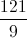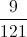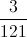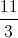Explanation: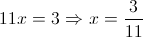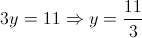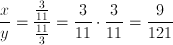### Example Question #4 : How To Multiply Fractions

At a certain company, one quarter of the employees take the bus to work and one third drive. Of the remaining employees, half walk, one third ride a bike, and the rest take the subway.

Out of the total number of employees, what fraction ride a bike to work?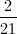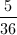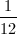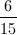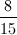Explanation:

First we want to find the fraction of employees that neither take the bus nor drive, so we’ll add the fractions that do take the bus or drive and subtract that result from the total.

Bus:Drive:Remaining: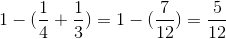Now we need the fraction representing one third of these remaining employees (the fraction that ride a bike). Since "of " means multiply, we'll multiply.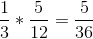### Example Question #5 : How To Multiply Fractions

Simplify: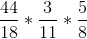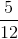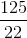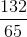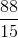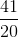Explanation:

Multiplying fractions is very easy. All you do is multiply all the numerators by each other and all the denominators by each other. You do not have to do anything that has to do with fancy common denominators like you do for adding and subtracting. For a question like this, it is often easiest just to cancel factors before you start your final multiplication. First, note: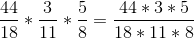Now, cancel thefrom the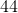: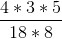Next, thein the numerator cancels with thein the denominator: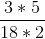Finally, thein the numerator cancels with thein the denominator: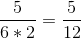### Example Question #6 : How To Multiply Fractions

Simplify: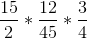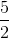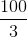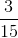Explanation:

Multiplying fractions is very easy. All you do is multiply all the numerators by each other and all the denominators by each other. You do not have to do anything that has to do with fancy common denominators like you do for adding and subtracting. For a question like this, it is often easiest just to cancel factors before you start your final multiplication. First, note: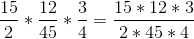Now, cancel thein the denominator with thein the numerator: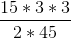Next, the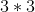in the numerator cancels with thein the denominator: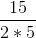Finally, cancel thein the denominator with thein the numerator:### Example Question #7 : How To Multiply Fractions

Solve for: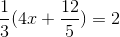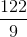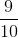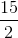Explanation:

Begin by distributing the group on the left side of the equation. Remember that it is easy to multiply fractions. You only need to multiply the denominators and numerators. There are no "fancy" steps in between.

Therefore,is the same as: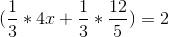You can cancel part of the second fraction out, so you get: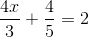Now, subtractfrom both sides: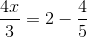Simplifying the right side of the equation, you get...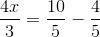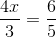Now, multiply both sides by: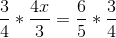Simplify: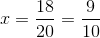Tired of practice problems?

Try live online GRE prep today.